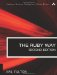# Section 5.22. Trigonometry in Degrees, Radians, and Grads

### 5.21. Numerical Computation of a Definite Integral

I'm very good at integral and differential calculus...

W. S. Gilbert, The Pirates of Penzance, Act 1

If you want to estimate the value of a definite integral, there is a time-tested technique for doing so. This is what the calculus student will remember as a Riemann sum.

The integrate method shown here takes beginning and ending values for the dependent variable as well as an increment. The fourth parameter (which is not really a parameter) is a block. This block should evaluate a function based on the value of the dependent variable passed into that block. (Here we are using "variable" in the mathematical sense, not in the computing sense.) It is not necessary to define a function to call in this block, but we do so here for clarity:

`def integrate(x0, x1, dx=(x1-x0)/1000.0)   x = x0   sum = 0   loop do     y = yield(x)     sum += dx * y     x += dx     break if x > x1   end   sum end def f(x)   x**2 end z = integrate(0.0,5.0) {|x| f(x) } puts z, "\n"           # 41.7291875`

Note that in the preceding example, we are relying on the fact that a block returns a value that yield can retrieve. We also make certain assumptions here. First, we assume that x0 is less than x1 (otherwise, an infinite loop results); the reader can easily improve the code in details such as this one. Second, we assume that the function can be evaluated at arbitrary points in the specified domain. If at any time we try to evaluate the function at such a point, chaos will ensue. (Such functions are generally not integrable anyway, at least over that set of x values. Consider the function f(x)=x/(x-3) when x is 3.)

Drawing on our faded memories of calculus, we might compute the result here to be 41.666 or thereabout (5 cubed divided by 3). Why is the answer not as exact as we might like? It is because of the size of the "slice" in the Riemann sum; a smaller value for dx will result in greater accuracy (at the expense of an increase in runtime).

Finally, we will point out that a function like this is more useful when we have a variety of functions of arbitrary complexity, not just a simple function like f(x) = x**2.The Ruby Way, Second Edition: Solutions and Techniques in Ruby Programming (2nd Edition)
ISBN: 0672328844
EAN: 2147483647
Year: 2004
Pages: 269
Authors: Hal Fulton

Similar book on Amazon

flylib.com © 2008-2017.
If you may any questions please contact us: flylib@qtcs.net